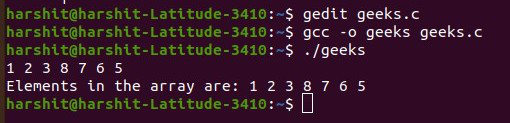# C program to input an array from a sequence of space-separated integers

• Difficulty Level : Easy
• Last Updated : 15 Jul, 2021

Given a string S consisting of space-separated integers, the task is to write a C program to take the integers as input from the string S and store them in an array arr[].

Examples:

Input: S = “1 2 3 4”
Output: {1, 2, 3, 4}

Input: S = “32 12”
Output: {32, 12}

Approach: The idea is to solve the given problem is to use getchar() function to check if a ‘\n’ (newline) occurs is found while taking input and then stop the input. Follow the step below to solve the given problem:

• Initialize a variable, say count, which is used to store the index of the array element.
• Initialize an array arr[] of size 106 to store the elements into the array.
• Iterate using a do-while loop until newLine occurs and perform the following steps:
• Store the current value at index count as scanf(“%d “, &arr[count]); and increment the value of count.
• If the next character is not endline, then continue. Otherwise, break out of the loop.
• After completing the above steps, print the elements stored in the array.

Below is the implementation of the above approach:

## C

 `// C program for the above approach``#include ` `// Driver Code``int` `main()``{``    ``// Stores the index where the``    ``// element is to be inserted``    ``int` `count = 0;` `    ``// Initialize an array``    ``int` `a;` `    ``// Perform a do-while loop``    ``do` `{` `        ``// Take input at position count``        ``// and increment count``        ``scanf``(``"%d"``, &a[count++]);` `        ``// If '\n' (newline) has occurred``        ``// or the whole array is filled,``        ``// then exit the loop` `        ``// Otherwise, continue``    ``} ``while` `(``getchar``() != ``'\n'` `&& count < 100);` `    ``// Resize the array size to count``    ``a[count];` `    ``// Print the array elements``    ``for` `(``int` `i = 0; i < count; i++) {``        ``printf``(``"%d, "``, a[i]);``    ``}` `    ``return` `0;``}`

Output:My Personal Notes arrow_drop_up